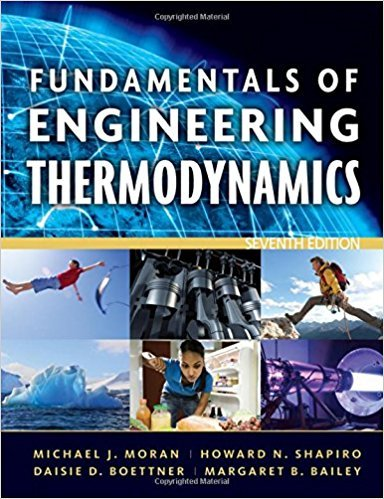×
Get Full Access to Fundamentals Of Engineering Thermodynamics - 7 Edition - Chapter 1 - Problem 55p
Get Full Access to Fundamentals Of Engineering Thermodynamics - 7 Edition - Chapter 1 - Problem 55p

×

# Exploring TemperatureFigure P1.55 shows a systemISBN: 9780470495902 50

## Solution for problem 55P Chapter 1

Fundamentals of Engineering Thermodynamics | 7th Edition

• Textbook Solutions
• 2901 Step-by-step solutions solved by professors and subject experts
• Get 24/7 help from StudySoup virtual teaching assistantsFundamentals of Engineering Thermodynamics | 7th Edition

4 5 1 287 Reviews
23
2
Problem 55P

Problem 55P

Exploring Temperature

Figure P1.55 shows a system consisting of a cylindrical copper rod insulated on its lateral surface while its ends are in contact with hot and cold walls at temperatures 1000°R and 500°R, respectively.

(a) Sketch the variation of temperature with position through the rod, x.

(b) Is the rod in equilibrium? Explain.Fig. P1.55

Step-by-Step Solution:

Step 1 of 4

We have to plot graph temperature(T) versus  distance (x)  and we need to explain whether the rod is in equilibrium or not.

Step 2 of 4

Step 3 of 4

##### ISBN: 9780470495902

Unlock Textbook Solution